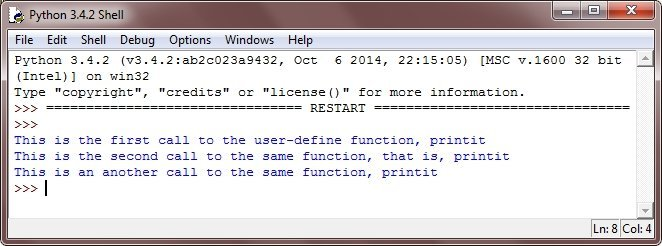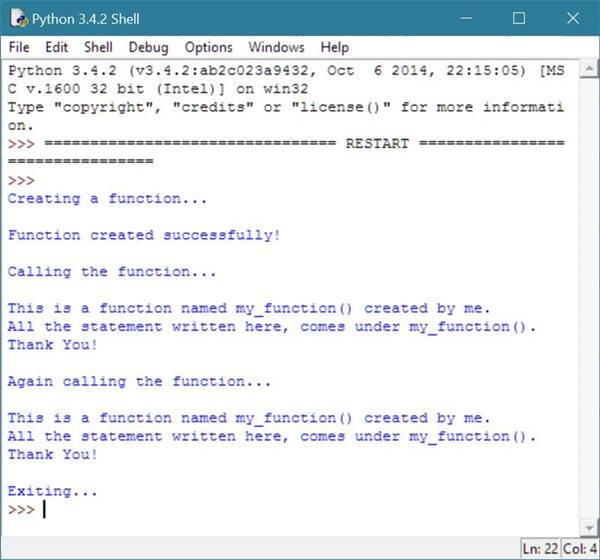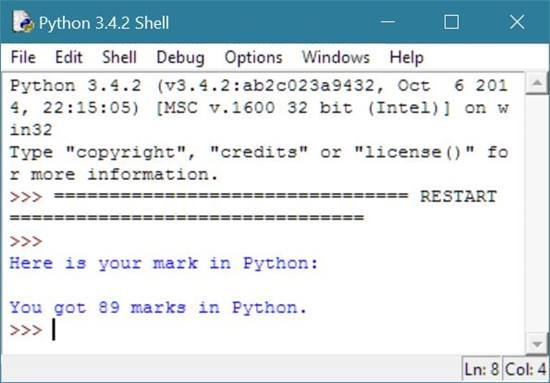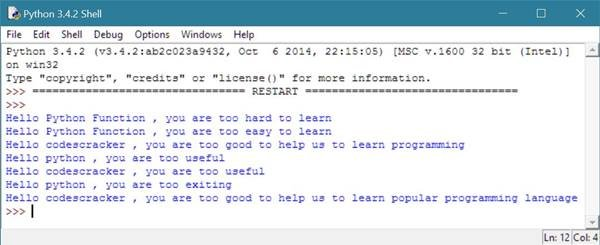# Python Functions

Here you will learn about functions in python, that is how to define a function, function calling, programmer-defined function, function with parameter, function with default argument or parameter value etc with examples.

A function in python, is a block of code, used to perform a particular action.

## Python Function Definition

To define a function in python, follow these rules:

• function blocks in python, begins with the keyword def, followed by the function name and then parentheses
• the parameters/arguments should be placed inside these parentheses
• the code block of the function starts with a colon (:)
• and now the return statement exits a function, passing back an expression to the function caller
• a return statement with no any arguments is the same as return None

Here is the general form to define a function in python:

```def function_name(parameters):
"function_doc_string"
function_suite
return [expression]```

Here is an example, takes one string parameter and prints this argument as output:

```def printit(strn):
"This will print the passed string into this function"
print(strn)
return```

## Python Function Calling

To execute a function in python, you have to call it from another function or directly from the python prompt. Here is an example, showing how to call a function in python:

```# Python Functions - Call a Function in Python - Example Program

def printit(strn):
"This will print a passed string inside this function"
print(strn)
return;

printit("This is the first call to the user-define function, printit");
printit("This is the second call to the same function, that is, printit");
printit("This is an another call to the same function, printit");```

Here is the output produced by the above python program:## Python Function Example

Let's take an example demonstrates functions in python. This example, demonstrates about how to create or define a function and how to call a function in python.

```print("Creating a function...\n");
def my_function():
print("This is a function named my_function() created by me.");
print("All the statement written here, comes under my_function().");
print("Thank You!");
print("Function created successfully!");
print("\nCalling the function...\n");
my_function();
print("\nAgain calling the function...\n");
my_function();
print("\nExiting...");```

Here is the sample output of the above example.## Python Function Parameters and Return Values

Here is an example illustrates about function parameters and return values in python.

```def display_your_marks(msg_abt_ur_mrks):
print(msg_abt_ur_mrks);
def your_marks():
marks = 89;
return marks;
display_your_marks("Here is your mark in Python:\n");
mark = your_marks();
print("You got",mark,"marks in Python.");```

Below is the screenshot of the sample output produced by above example.As you can see from the above example, msg_abt_ur_mrks is the parameter of the function display_your_marks() and marks comes after the return keyword in the function, your_marks() is the return value, that contains 89 at the moment.

## Python Function Default Parameter Values

Let's take an example that demonstrates about default parameter values of a python function.

```def my_function_one(topic, phrase):
print("Hello",topic,", you are too",phrase);

# now let's create another function with default parameters
def my_function_two(topic = "python", phrase = "useful"):
print("Hello",topic,", you are too",phrase);

# now let's call the above two functions in different-different ways
my_function_one("Python Function", "hard to learn");
my_function_one(topic = "Python Function", phrase = "easy to learn");
my_function_one(phrase = "good to help us to learn programming", topic = "codescracker");
my_function_two();
my_function_two(topic = "codescracker");
my_function_two(phrase = "exiting");
my_function_two("codescracker", "good to help us to learn popular programming language");```

Here is the screenshot of the sample output produced by the above example of default argument or parameter values in python function.As you can see from the above example, there are two function defined here, namely, my_function_one() and my_function_two(). In these two functions, my_function_two() is the function that contains two default parameters with values, python and useful, that is, if you will not provide any values to these two parameter, then the default value will be used as shown in the above example.

## Python Type Conversion Functions

The table given below list type conversion functions available in python with its short description.

Function Description
int(v) returns an integer value
float(v) returns a floating-point value
str(v) returns a string value

Tools
Calculator